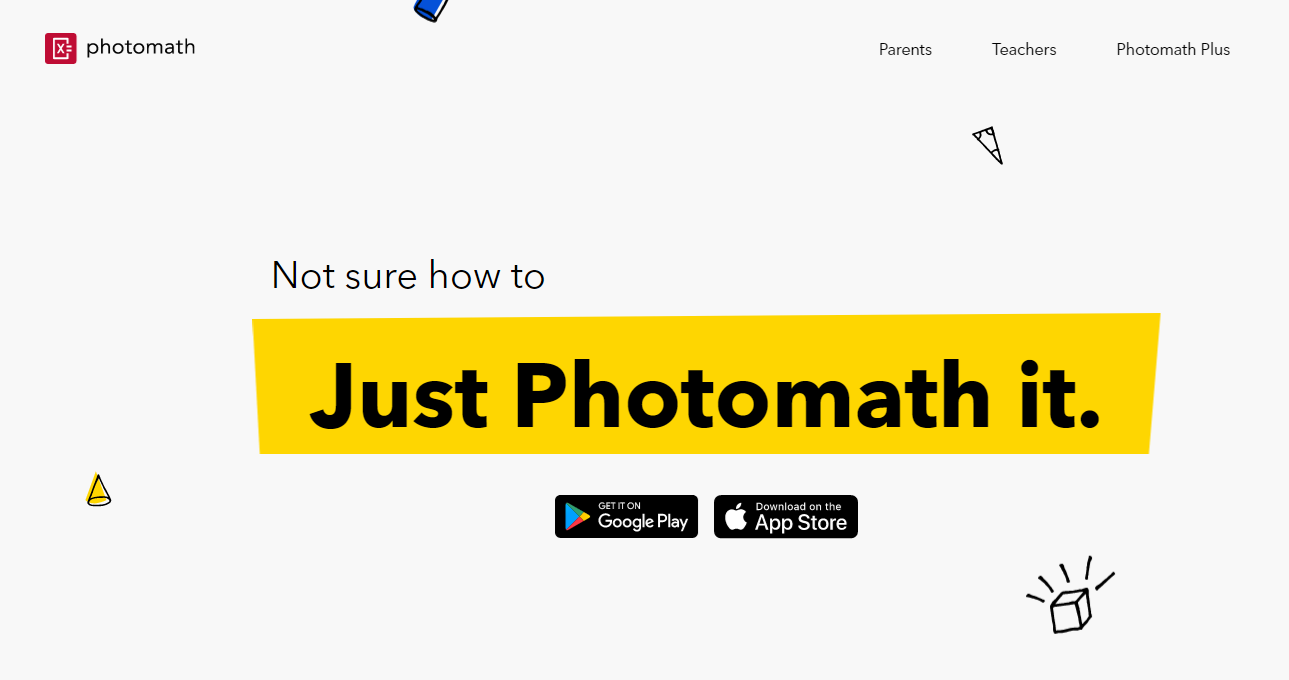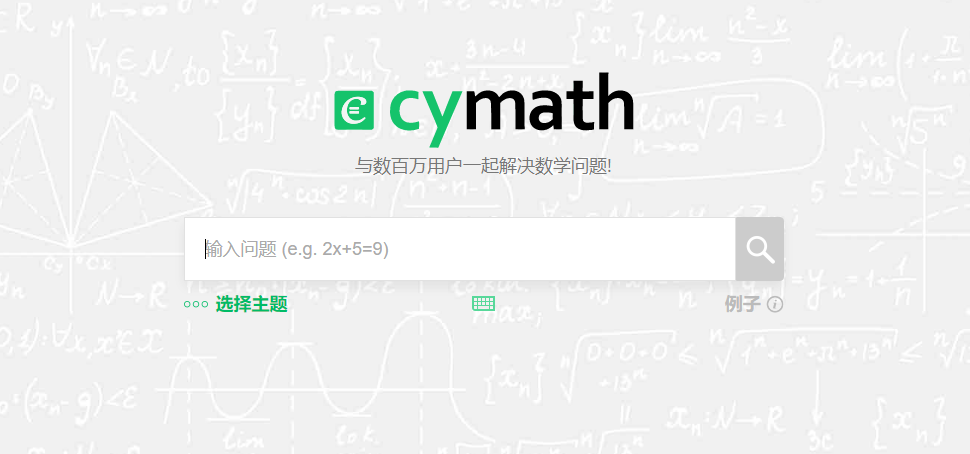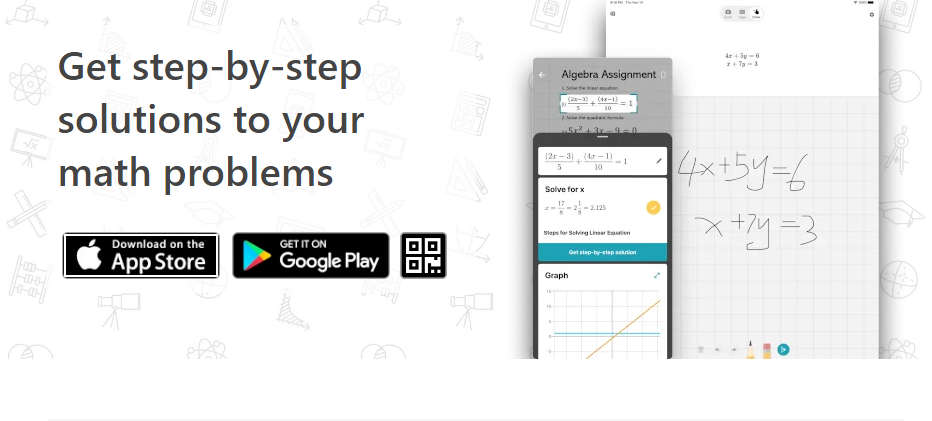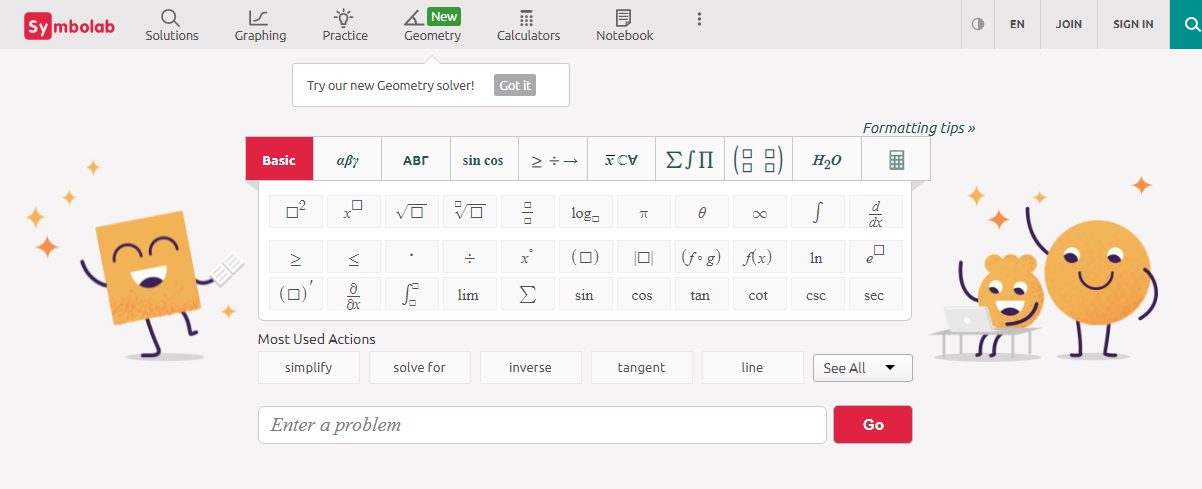# 5 Best Math Solver AppsThe use of technology has raised drastically. More students than ever now use technology in their studying than ever. Smartphone tech is one of the prior parts of this scenario. From searching online to creating homework copies smartphones can help students in many ways. One of the latest addition to these ways is a math solver app. Also known as photo math apps, these apps help students to solve their math problems just by clicking a photo of the problem. Math solver apps can solve arithmetic, fractions, linear equations, Geometry, and many other types of maths.

## 5 Best Math Solver Apps

There are many math solver apps available for smartphones. They have diverse functionalities and features. Here we have listed the best photo math apps with their features, how to use them, what maths they can do, compatibility and pricing. Let's take a look at the list.

1. Photomath
2. Cymath - Math Problem Solver
3. Mathway: Scan & Solve Problems
4. Microsoft Math Solver
5. Symbolab

### 1. PhotomathStarting with the Photomath app. This app can do math just by scanning and also explains the problem. This way it helps students and others solve math problems. The Photomath app provides Step-by-step explanations for every solution to help you learn maths easily and fast. It also works as a Multi-functional scientific calculator. Photomath can solve Basic Math, Algebra, Geometry, Calculus, Statistics. The best thing about the app is the detailed animations that help you visualize and understand each math step. Photomath app starts free. Premium subscription costs \$5 per month(billed annually). It is available for Android and iOS.

### 2. Cymath - Math Problem SolverCymath - Math Problem Solver is another popular app for solving math problems. This solver has a math engine that lets you solve problems on the go. This math problem solver is designed to help you with your math homework. This photomath app also shows you how to get there step-by-step. This app can do partial fraction, polynomial division, integration by parts, integration by partial fraction, trigonometric substitution, and more. The best thing about this app is that it has a handwriting option and can solve problems by handwriting. Cymath is a free app. It is available for iOS and Android. Cymath is one of the most popular math-solving websites.

### 3. Mathway: Scan & Solve ProblemsMathway is the world’s smartest math calculator for algebra, graphing, calculus, and more! Mathway gives you unlimited access to math solutions that can help you understand complex concepts. Simply point your camera and snap a photo or type your math homework question for step-by-step answers. This app helps a lot when you are working with algebra. It gives you straight answers to your math problems, at times. Mathway is the best free calculator that explains answers. This app is simple and easy to use. It is a free app. Mathway is available for iOS and Android.

### 4. Microsoft Math SolverNext comes the Microsoft Math Solver app. This is an app for younger math heads. This AI-powered math solver can do a lot. You can use this photomath app both by writing down a math problem or by taking a picture of your problem. The math solver can recognize the math photo and it then solves the problem for you. This math solver can solve Elementary, Pre-Algebra, Algebra, Word problems on mathematical concepts, number theory, probability, volume, surface area, and more. The best thing about this app is that it lets you learn math in your language. It supports many languages including Chinese, French, German, Hindi, Italian, Japanese, Portuguese, Russian, Spanish, and many more. This is a free app. It is available for iOS and Android.

### 5. Symbolab - Math solverSymbolab Math Solver solves any math problem including Pre- Algebra, Algebra, Pre-Calculus, Calculus, Trigonometry, Functions, Matrix, Vectors, Geometry, and Statistics. Many students use this app to understand math problems and explanations. This app can do Graphing Calculator, Integral Calculator, Inequality Calculator, Functions Calculator, and more. The best thing about this photomath app is that helps teach different math techniques. This app can solve sums quickly and is easy to use. Symbolab starts free. It also offers premium subscriptions for advanced features. It is available for Android and iOS.

Thanks for reading the 5 Best Math Solver Apps list. Share it with your friends.

5 Must Have Math Apps For Students